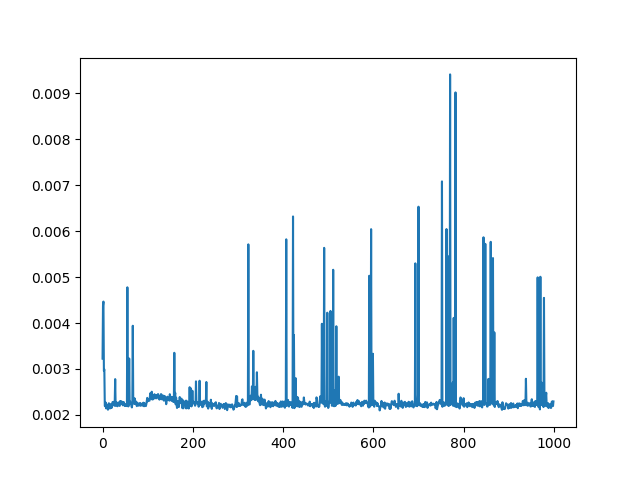# torch.Tensor() sometimes (inconsistently) slow

Hello,
I have a video procesing pipeline that reads frames from video and feeds them to pytorch model. I observed that the call of torch.Tensor() sometimes takes significantly more time than expected.

The pipeline can be simulated by script like this:

``````import torch
import numpy as np
import cv2
from time import time
import matplotlib.pyplot as plt

video = cv2.VideoCapture("video.mp4")

processing_times = []

for i in range(1000):
_, frame = video.read() # each frame is numpy array of shape (1080,1920,3)
frame = np.copy(frame)

t_start = time()
x = torch.Tensor(frame)
t_end = time()

processing_times.append(t_end - t_start)

plt.plot(processing_times)
plt.savefig('plot.png')
video.release()
``````

Note that the time is measured only on the call of torch.Tensor(frame) and does not include reading the frame from video.
The plot from the script looks like this:So the call of torch.Tensor(frame) takes usually about 25ms, but sometimes spikes up to the 90 ms.
Also, the call time seems to be random, when I re-run the script, the graph looks differently (so it’s not the particular frames that causes the long call time).
Sometimes, when re-running the script, the plot is almost withou any spikes (the call time is always between 20-30ms).

My system:
Ubuntu 19.10
Python 3.7
Torch 1.5.1

I run the script from terminal without IDE (to avoid some garbage that may cause the long call time).

So I have no idea what may cause the call of torch.Tensor() to take sometimes much longer time thats usual. I would be grateful for any advices / comments.

edit: I observed that removing the
`frame = np.copy(frame)`
line seems to reduce the problem, the number of spikes is then much lower (although it still may happen that one call takes about 90 ms). The PyTorch tensors share the memory buffer of NumPy ndarrays which may be part of the problem, hovewer I still don’t see how this translates to the sometimes longer torch.Tensor() call?

-Jakub

This shouldn’t be the case in your code snippet, as you are creating a new tensor and thus trigger a copy.
To share the same underlying data, you would have to use `torch.from_numpy` as seen here:

``````frame = np.ones((2, 2))
x = torch.Tensor(frame)

x[0, 0] = 2.
print(x)
> tensor([[2., 1.],
[1., 1.]])
print(frame)
> [[1. 1.]
[1. 1.]]

y = torch.from_numpy(frame)
y[0, 0] = 2.
print(y)
> tensor([[2., 1.],
[1., 1.]], dtype=torch.float64)
print(frame)
> [[2. 1.]
[1. 1.]]
``````